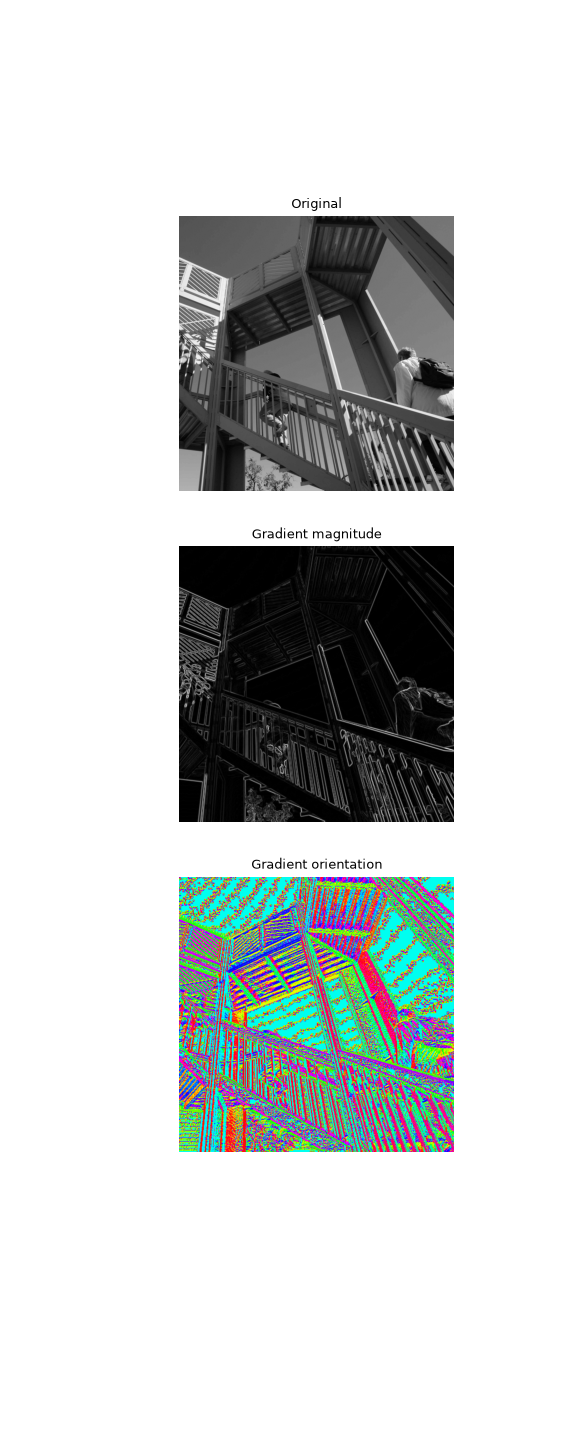# scipy.signal.convolve2d¶

scipy.signal.convolve2d(in1, in2, mode='full', boundary='fill', fillvalue=0)[source]

Convolve two 2-dimensional arrays.

Convolve in1 and in2 with output size determined by mode, and boundary conditions determined by boundary and fillvalue.

Parameters
in1array_like

First input.

in2array_like

Second input. Should have the same number of dimensions as in1.

modestr {‘full’, ‘valid’, ‘same’}, optional

A string indicating the size of the output:

`full`

The output is the full discrete linear convolution of the inputs. (Default)

`valid`

The output consists only of those elements that do not rely on the zero-padding. In ‘valid’ mode, either in1 or in2 must be at least as large as the other in every dimension.

`same`

The output is the same size as in1, centered with respect to the ‘full’ output.

boundarystr {‘fill’, ‘wrap’, ‘symm’}, optional

A flag indicating how to handle boundaries:

`fill`

pad input arrays with fillvalue. (default)

`wrap`

circular boundary conditions.

`symm`

symmetrical boundary conditions.

fillvaluescalar, optional

Value to fill pad input arrays with. Default is 0.

Returns
outndarray

A 2-dimensional array containing a subset of the discrete linear convolution of in1 with in2.

Examples

Compute the gradient of an image by 2D convolution with a complex Scharr operator. (Horizontal operator is real, vertical is imaginary.) Use symmetric boundary condition to avoid creating edges at the image boundaries.

```>>> from scipy import signal
>>> from scipy import misc
>>> ascent = misc.ascent()
>>> scharr = np.array([[ -3-3j, 0-10j,  +3 -3j],
...                    [-10+0j, 0+ 0j, +10 +0j],
...                    [ -3+3j, 0+10j,  +3 +3j]]) # Gx + j*Gy
>>> grad = signal.convolve2d(ascent, scharr, boundary='symm', mode='same')
```
```>>> import matplotlib.pyplot as plt
>>> fig, (ax_orig, ax_mag, ax_ang) = plt.subplots(3, 1, figsize=(6, 15))
>>> ax_orig.imshow(ascent, cmap='gray')
>>> ax_orig.set_title('Original')
>>> ax_orig.set_axis_off()